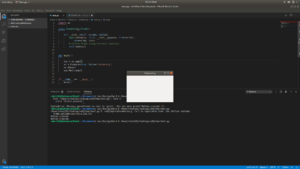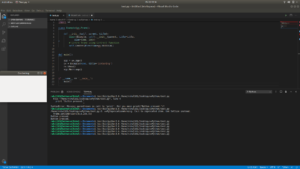Related Articles
wxPython – Set window in center of screen
• Last Updated : 10 May, 2020

In this article we are going to learn that, how can we show window in the center of the screen. We can do this by using Centre() function in `wx.Frame `module.

Syntax:

```wx.Frame.Centre(self, direction = wx.BOTH)
```

Parmeters:

ParameterInput TypeDescription
directionintThe parameter may be wx.HORIZONTAL, wx.VERTICAL or wx.BOTH.

Example #1:

 `# import wxPython``import` `wx`` ` `class` `Example(wx.Frame):`` ` `    ``def` `__init__(``self``, parent, title):`` ` `        ``super``(Example, ``self``).__init__(parent, title ``=` `title,``                                           ``size ``=``(``300``, ``200``))`` ` `        ``# Centre frame using Centre() function``        ``self``.Centre()`` ` `def` `main():`` ` `    ``app ``=` `wx.App()``    ``ex ``=` `Example(``None``, title ``=``'Centering'``)``    ``ex.Show()``    ``app.MainLoop()`` ` ` ` `if` `__name__ ``=``=` `'__main__'``:``    ``main()`

Output:Example #2:

 `# import wxPython``import` `wx`` ` `class` `Example(wx.Frame):`` ` `    ``def` `__init__(``self``, parent, title):``        ``super``(Example, ``self``).__init__(parent, title ``=` `title,``                                          ``size ``=``(``300``, ``200``))`` ` `        ``# Centre frame using Centre() function``        ``self``.Centre(direction ``=` `wx.VERTICAL)`` ` ` ` `def` `main():`` ` `    ``app ``=` `wx.App()``    ``ex ``=` `Example(``None``, title ``=``'Centering'``)``    ``ex.Show()``    ``app.MainLoop()`` ` ` ` `if` `__name__ ``=``=` `'__main__'``:``    ``main()`

Output:Attention geek! Strengthen your foundations with the Python Programming Foundation Course and learn the basics.

To begin with, your interview preparations Enhance your Data Structures concepts with the Python DS Course. And to begin with your Machine Learning Journey, join the Machine Learning – Basic Level Course

My Personal Notes arrow_drop_up